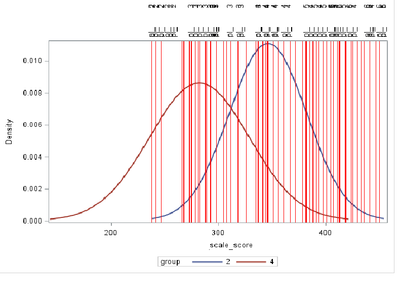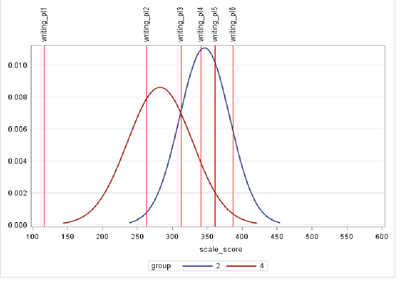## Reference lines by a macro

Hi all,

I hope I can explain well what I need. I have a data set as provided below.

1 130 2

6 124 4

1 156 2

3 234 1

2 256 4

12 300 2

...

I want to draw an overlayed distribution with reference lines for groups 2 and 4 per 12 grades. I can use that code:

proc sgplot data=sample;
where group in ("2" , "4"); /* restrict to two groups */
density ss/ type=normal group=group; /* overlay density estimates */
refline 116 / LABEL="ref1" lineattrs=(color=red) axis=x discreteoffset=0.5;

refline 223 / LABEL="ref2" lineattrs=(color=red) axis=x discreteoffset=0.5;

But the problem is that I have 12 grades and each grade's reference lines are different. For example for grade 1 ref1=116, ref2=223 and for grade2 ref1=118, ref2=245. I have another data set as below that has those reference lines. As you see that the reference line is the first score of each ref variable per grade.

1 116 1

1 118 1

1 223 2

1 224 2

1 245 2

2 118 1

2 131 1

2 245 2

2 246 2

So, it there a way to do this with a macro?

Thank you,

3 REPLIES 3

## Re: Reference lines by a macro

So you need to create a series of statements like this:

``refline 116 / LABEL="ref1" lineattrs=(color=red) axis=x discreteoffset=0.5;``

populated from the first values of ref within the grades?

I would use CALL EXECUTE to create the code:

``````data _null_;
set other_dataset end=done;
if _n_ = 1 then call execute('
proc sgplot data=sample;
where group in ("2" , "4"); /* restrict to two groups */
density ss/ type=normal group=group;
');
if first.ref then call execute('
refline ' !! put(score,best.) !! ' / LABEL="ref' !! trim(put(ref,best.)) !! '" lineattrs=(color=red) axis=x discreteoffset=0.5;
');
if done then call execute('run;');
run;``````

## Re: Reference lines by a macro

Thank you for your help. Here is what I got from the code that you provided. It is working but it's not drawing distributions per grade and it has so many lines. However, I should have only 6 lines per grade.And I should get something like below per grade.Thank you,

## Re: Reference lines by a macro

Please post the data (in data steps like this:

``````data sample;
datalines;
1 130 2
6 124 4
1 156 2
3 234 1
2 256 4
12 300 2
;

data other_dataset;
datalines;
1 116 1
1 118 1
1 223 2
1 224 2
1 245 2
2 118 1
2 131 1
2 245 2
2 246 2
;``````

) and the code that created the second graph.

Don't forget to add the dataset you intend to use for making the code dynamic.

Macro development starts with working code without macro elements.

Discussion stats
• 3 replies
• 397 views
• 0 likes
• 2 in conversation